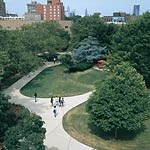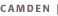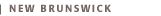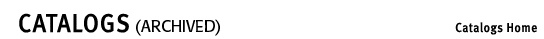# Applied and Computational Mathematics OptionThe applied and computational mathematics track is designed for students with interests in mathematics and computer science. Students are taught mathematical ideas and methods useful for solving computational problems in science and engineering. Students majoring in computer science, or in other scientific disciplines, who have a strong interest in mathematics are encouraged to pursue a second major in applied and computational mathematics.First Year50:640:121  Unified Calculus I (4)50:640:122  Unified Calculus II (4)50:750:131, 133  Elements of Physics I, Elements of Physics Laboratory I (3,1)50:750:132, 134  Elements of Physics II, Elements of Physics Laboratory II (3,1)Sophomore Year50:198:111,112  Programming Fundamentals, Software Laboratory I (3,1)50:640:221  Unified Calculus III (4)50:640:237  Discrete Mathematics (3)50:640:250  Linear Algebra (3)Junior Year50:198:113,114  Object-Oriented Programming, Software Laboratory II (3,1)50:198:371  Design and Analysis of Algorithms (3)50:198:381  Introduction to Numerical Methods (3)50:640:314  Elementary Differential Equations (3)Senior Year50:198:481  Advanced Numerical Methods (3)50:640:358  Advanced Discrete Mathematics (3)50:640:463  Partial Differential Equations and Boundary Value Problems (3)Applied and computational mathematics elective (3)Elective Courses50:198:316  Parallel Programming (3)50:198:456  Computer Graphics (3)50:198:458  Scientific Visualization (3)50:640:345  Mathematics on the Web (3)50:640:347  Visualizing Mathematics by Computer (3)50:640:357  Computational Mathematics (3)50:640:427  Advanced Differential Equations (3)56:645:557  Signal Processing (3)56:645:558  Queuing Theory (3)For a minor in computer science, a student needs to take the following additional two courses:50:198:231  Introduction to Computer Organization (3)50:198:321  Programming Language Concepts (3)